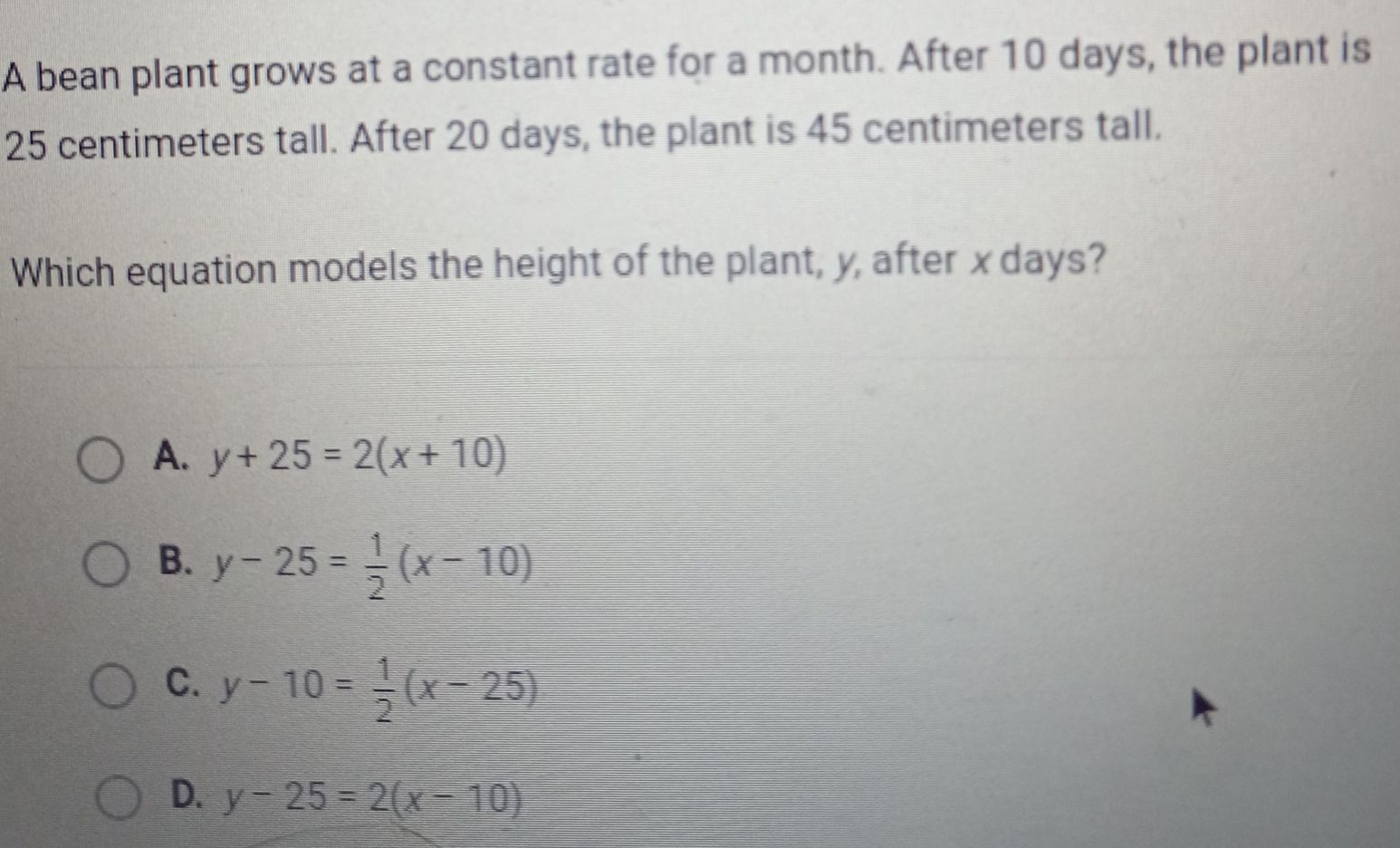### ¿Todavía tienes preguntas de matemáticas?

Pregunte a nuestros tutores expertos
Algebra
PreguntaA bean plant grows at a constant rate for a month. After $$10$$ days, the plant is $$25$$ centimeters tall. After $$20$$ days, the plant is $$45$$ centimeters tall. Which equation models the height of the plant, $$y$$ , after $$x$$ days?

A. $$y + 25 = 2 ( x + 10 )$$

B. $$y - 25 = \frac { 1 } { 2 } ( x - 10 )$$

C. $$y - 10 = \frac { 1 } { 2 } ( x - 25 )$$

D. $$y - 25 = 2 ( x - 10 )$$